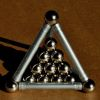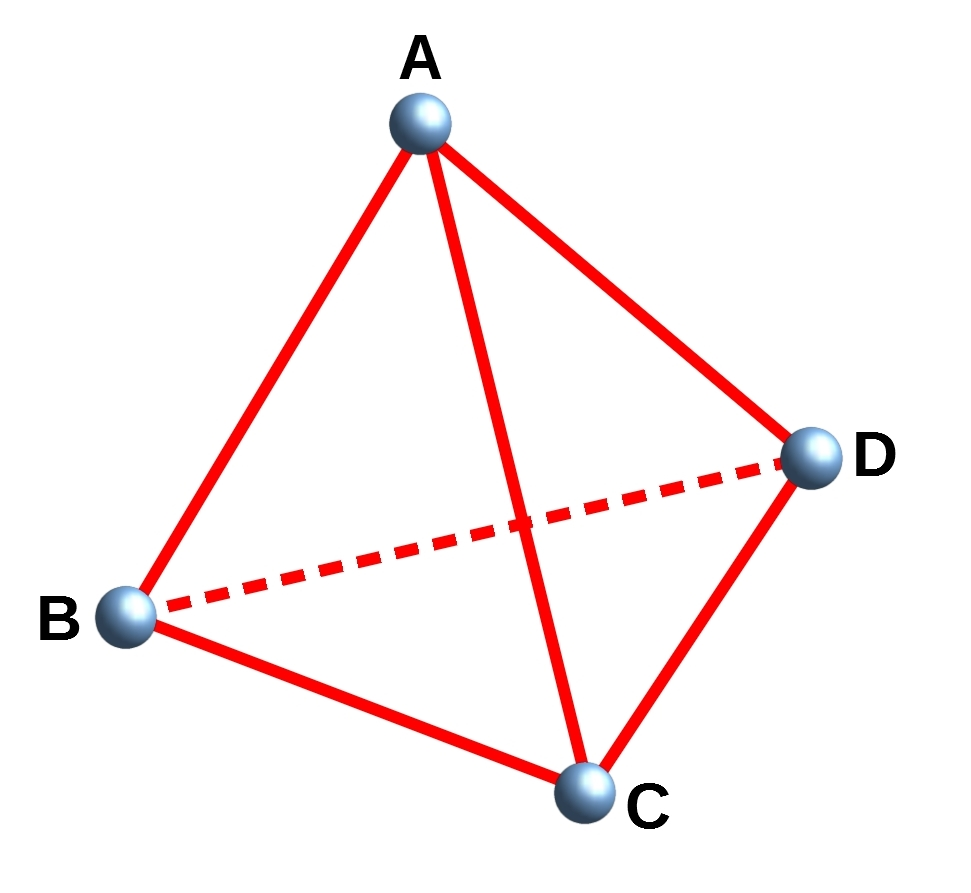#### You may also like### Reach for Polydron

A tetrahedron has two identical equilateral triangles faces, of side length 1 unit. The other two faces are right angled isosceles triangles. Find the exact volume of the tetrahedron.### Tetra Inequalities

Can you prove that in every tetrahedron there is a vertex where the three edges meeting at that vertex have lengths which could be the sides of a triangle?### Tetra Square

ABCD is a regular tetrahedron and the points P, Q, R and S are the midpoints of the edges AB, BD, CD and CA. Prove that PQRS is a square.

# Tetra Perp

##### Age 16 to 18Challenge Level

A tetrahedron $ABCD$ has vertices $A$, $B$, $C$ and $D$, as shown below:Show that the edges $AD$ and $BC$ of the tetrahedron are mutually perpendicular if and only if $AB^2+CD^2 = AC^2+BD^2$.

If the position vector of $A$ is ${\bf a}$ (and similar for the other vertices), can you use the scalar product to find an expression for $AB^2$?

It might be helpful to note that $AB^2=|\overrightarrow{AB}|^2$.

Sometimes it is easier to try and show $p-q=0$ rather than trying to show $p=q$.